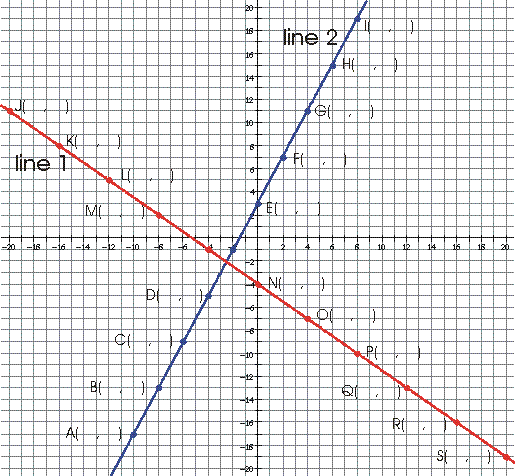Name:_____________________________________Date:__________________Per:______

Is the slope of a line independent of the points used to find the slope? If you find the slope from point A to point B on line 2 is it the same as the slope from point B to point A? What about the slope from point C to point F, is it the same as the slope from point A to point B? When finding the slope of a line does it matter which points you use? Does it matter which point you start with and which one you end with?1. Fill in the coordinates for each point that is labeled. If the point is a y-intercept circle it.
2. Fill in the slope (rise over run) for each pair of points below, use slope triangles to find the slope.

 Slope from A to B = Slope from E to D = Slope from P to L = Slope from B to A = Slope from G to C = Slope from L to P = Slope from A to E = Slope from B to F = Slope from K to R = Slope from D to H = Slope from J to L = Slope from R to K = Slope from I to C = Slope from N to O = Slope from K to J = Slope from F to B = Slope from P to S = Slope from S to Q =